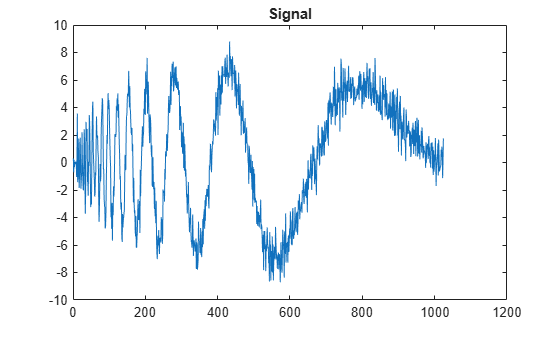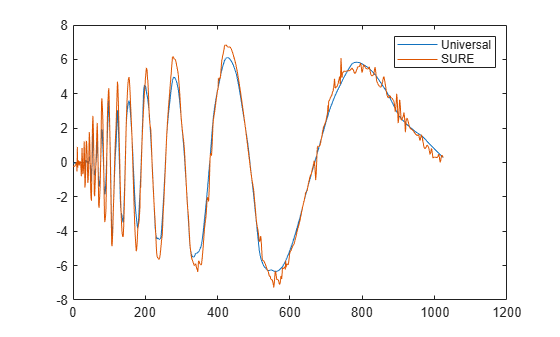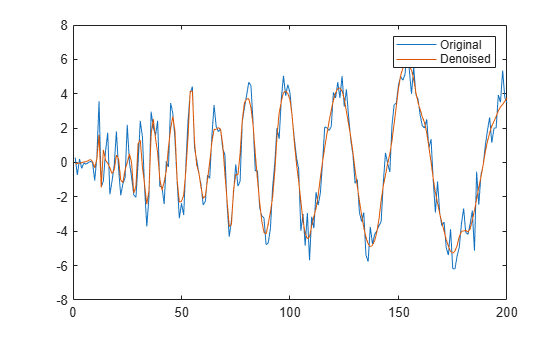Denoise Signal Using Generated C Code

This example shows how to denoise a signal using C code generated from the wdenoise function. Two techniques are demonstrated. The first technique uses a wrapper function which calls wdenoise. The second technique generates C code directly from wdenoise. Depending on your workflow, one technique may be preferred over the other. For example, to streamline code generation for large MATLAB® code, you can use wrapper functions to modularize the code.

Use Wrapper Function

Create a MATLAB function denoiseSignal that executes wdenoise and returns a denoised signal. The function takes two input arguments: a 1-D signal, and a denoising method. It passes these arguments to wdenoise. The function is included in the directory containing this example. The code for denoiseSignal follows.

function out = denoiseSignal(input,denMthd)
%#codegen
out = wdenoise(input,'DenoisingMethod',denMthd);
end

The %#codegen directive indicates that the function is intended for code generation.

Use codegen (MATLAB Coder) to generate a MEX function. For faster compilation, enable debug mode by setting the -g option. (In debug mode, the compiler disables some optimizations.) Code generation defaults to MEX code generation when you do not specify a build target. By default, codegen names the generated MEX function denoiseSignal_mex. To allow the generation of MEX file, specify the properties (class, size, and complexity) of the two input parameters:

• coder.typeof(0,[1 Inf]) specifies a row vector of arbitrary length containing real double values.

• coder.typeof('c',[1 Inf]) specifies a character array of arbitrary length.

codegen -g denoiseSignal -args {coder.typeof(0,[1 Inf]),coder.typeof('c',[1 Inf])}
Code generation successful.

plot(noisdopp)
title('Signal')Denoise the signal twice using denoiseSignal_mex. First, use the denoising method UniversalThreshold. Then, use the SURE method. Plot both results.

dn = denoiseSignal_mex(noisdopp,'UniversalThreshold');
dn2 = denoiseSignal_mex(noisdopp,'SURE');
plot([dn' dn2'])
legend('Universal','SURE')Use the MATLAB function and MEX function to denoise the signal using the Minimax method. Confirm the results are equal.

dnA = denoiseSignal_mex(noisdopp,'Minimax');
dnB = denoiseSignal(noisdopp,'Minimax');
max(abs(dnA-dnB))
ans = 6.2172e-15

Use Explicitly

You can also generate C code directly from wdenoise. Generate a MEX file that denoises a signal using the db4 wavelet. The generated MEX file is called wdenoise_mex.

codegen -g wdenoise -args {coder.typeof(0,[1 Inf]),coder.Constant('Wavelet'),coder.Constant('db4')}
Code generation successful.

Denoise the original signal.

dn3 = wdenoise_mex(noisdopp,'Wavelet','db4');

To confirm the MEX file accepts variable length inputs, denoise the first 200 samples of the original signal.

dn4 = wdenoise_mex(noisdopp(1:200),'Wavelet','db4');
plot([noisdopp(1:200)' dn4'])
legend('Original','Denoised')